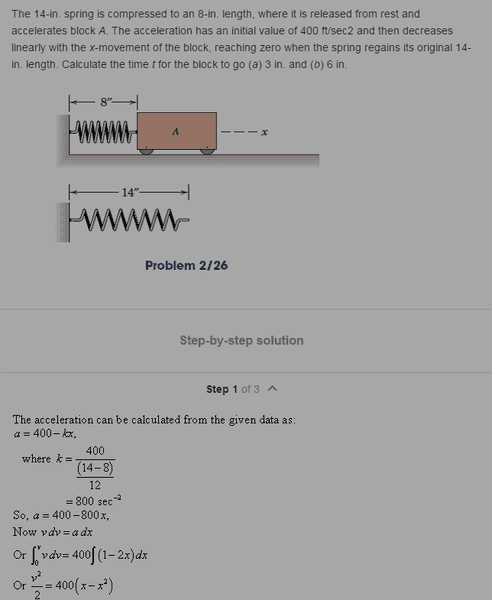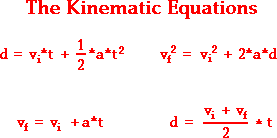# Spring Dynamic Kinematics - acceleration

## Homework Statement## Homework Equations

Standard physics equations before forces are introduced into kinematics## The Attempt at a Solution

I simply looked at Chegg and could not figure out how

a = 400 - kx

when logic dictates that

a = 400 - kx/m

, since this is possibly a sum of forces. Otherwise, it is lost to me how

F= -kx

is suddenly an acceleration quantity. Could someone explain that one line? Everything makes sense save for how

kx

stands alone in an acceleration equation. A classmate of mine who took this class before explained to me that in my dynamics class, mass is dropped from the equation when mass is not relevant to the question. However, he could not describe the reasoning behind it, so I was left somewhat unsatisfied by the answer.[/B]

•